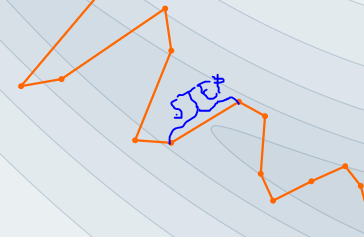# Effective value of LR

There is one old and dusty article in here: https://distill.pub/2017/momentum/And I think the alpha and beta are lr and momentum that corresponds to PyTorch:

`````` optimizer = torch.optim.SGD(model.parameters(), lr=0.05, momentum=0.9)
``````

Now, if I print the optimizer I will get:

SGD (
Parameter Group 0
dampening: 0
lr: 0.05
momentum: 0.9
nesterov: False
weight_decay: 0
)

But what I would like is to get the effective learning rate (ELR) inside the forward.
For instance if batchnumer=0 is the first batch, I assume ELR is 0.05.
As the batches counter increases ELR may change. See how in that article step size (ELR) will vary in size.

Can you give me the idea how to do that?

I assume this is one step (ELR):